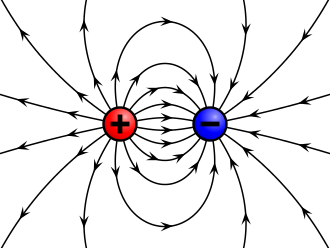# Interactions between chargesTwo charges $q_1$ and $q_2$ are separated by a certain distance, and they experience a force of $36\, \mu\text N$. If the distance between them is increased by $5\text{ m},$ the force becomes $25\, \mu\text{N}$.

Find the initial distance between the two charges (in meters).

×